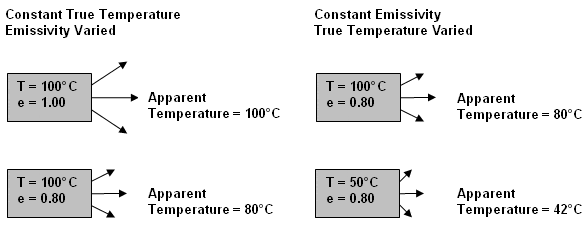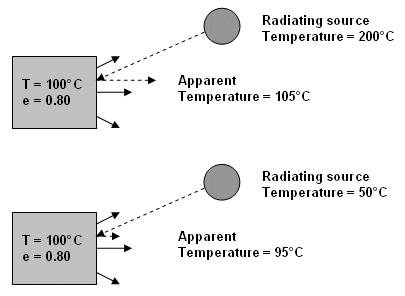+353 85 812 0054 [email protected]

### Effects of Emissivity

Thermal imaging cameras detect and measure the sum of infrared energy over a range of wavelengths determined by the sensitivity of the camera’s detector. Thermal imagers cannot discriminate energy at 7µm from energy at 14µm the way the human eye can distinguish various wavelengths of light as colors. They calculate the temperature objects by detecting and quantifying the emitted energy over the operational wavelength range of the detector. Temperature is then calculated by relating the measured energy to the temperature of a blackbody radiating an equivalent amount of energy according to Planck’s Blackbody Law.

Because the emissivity of an object affects how much energy an object emits, emissivity also influences a thermal imager’s temperature calculation. Consider the case of two objects at the same temperature, one having high emissivity and the other low. Even though the two objects have the same temperature, the one with the low emissivity will radiate less energy. Consequently, the temperature calculated by the thermal imager will be lower than that calculated for the high emissivity object.

#### Apparent Temperature

Thermal imaging cameras cannot detect the emissivity of objects in order to calculate their true temperature. They can only calculate the “apparent” temperature of objects. The apparent temperature of an object is a function of both its temperature and emissivity. Given two objects with the same true temperature but different emissivity, a higher apparent temperature will be calculated for the object with higher emissivity (see figure below). Given two objects with the same emissivity but different true temperature, a higher apparent temperature will be calculated for the object with higher true temperature. The apparent temperature of an object may be substantially different from its true temperature. Only when the emissivity of objects is known can thermal imagers compensate for emissivity and calculate true temperature.#### Reflectivity

Objects with high reflectivity can reflect energy radiated by other objects. For example, polished aluminum reflects about 90% of the energy incident upon its surface. Just as thermal imagers cannot detect the emissivity of objects in order to calculate their true temperature, they also can’t detect the reflectivity of objects. Therefore, when calculating the apparent temperature of an object, thermal imagers detect and quantify energy emitted from the object, as well as, energy reflected from the surface of the object. If an object reflects energy from another radiating source with a higher temperature, the apparent temperature that is calculated for the object will be higher than its true temperature. Likewise, if an object reflects energy from another radiating source with a lower temperature, the apparent temperature that is calculated for the object will be lower than its true temperature.

Given two objects with the same true temperature but different emissivity, a higher apparent temperature will be calculated for the object with higher emissivity (see figure below). Given two objects with the same emissivity but different true temperature, a higher apparent temperature will be calculated for the object with higher true temperature. The apparent temperature of an object may be substantially different from its true temperature. Only when the emissivity of objects is known can thermal imagers compensate for emissivity and calculate true temperature.